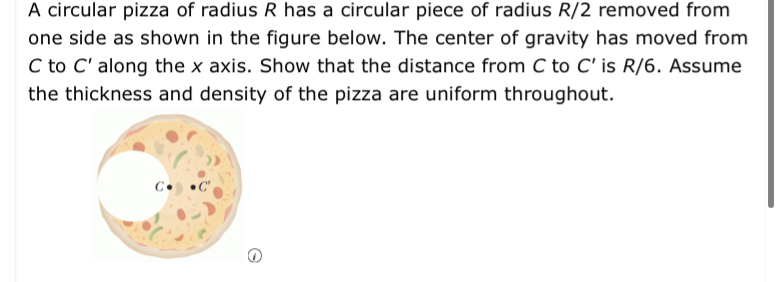# A circular pizza of radius R has a circular piece of radius R/2 removed fromone side as shown in the figure below. The center of gravity has moved fromC to C' along the x axis. Show that the distance from C to C' is R/6. Assumethe thickness and density of the pizza are uniform throughoutC

Question
25 viewshelp_outlineImage TranscriptioncloseA circular pizza of radius R has a circular piece of radius R/2 removed from one side as shown in the figure below. The center of gravity has moved from C to C' along the x axis. Show that the distance from C to C' is R/6. Assume the thickness and density of the pizza are uniform throughout C fullscreen
check_circle

Step 1

Write the expression for ...help_outlineImage TranscriptioncloseConsider the mass of the circular pizza of radius R is M, and the mass of R is m circular pizza having radius 2 The distance between C and C' is given by Мi — тх, М-т The mass of the circular pizza of radius R is given by Μ=ρ(TR)  here tis the thickness] is given by 2 The mass of the circular pizza of radius -A R χρt 2  m T fullscreen

### Want to see the full answer?

See Solution

#### Want to see this answer and more?

Solutions are written by subject experts who are available 24/7. Questions are typically answered within 1 hour.*

See Solution
*Response times may vary by subject and question.
Tagged in

### Centre of Mass and Centre of Gravity